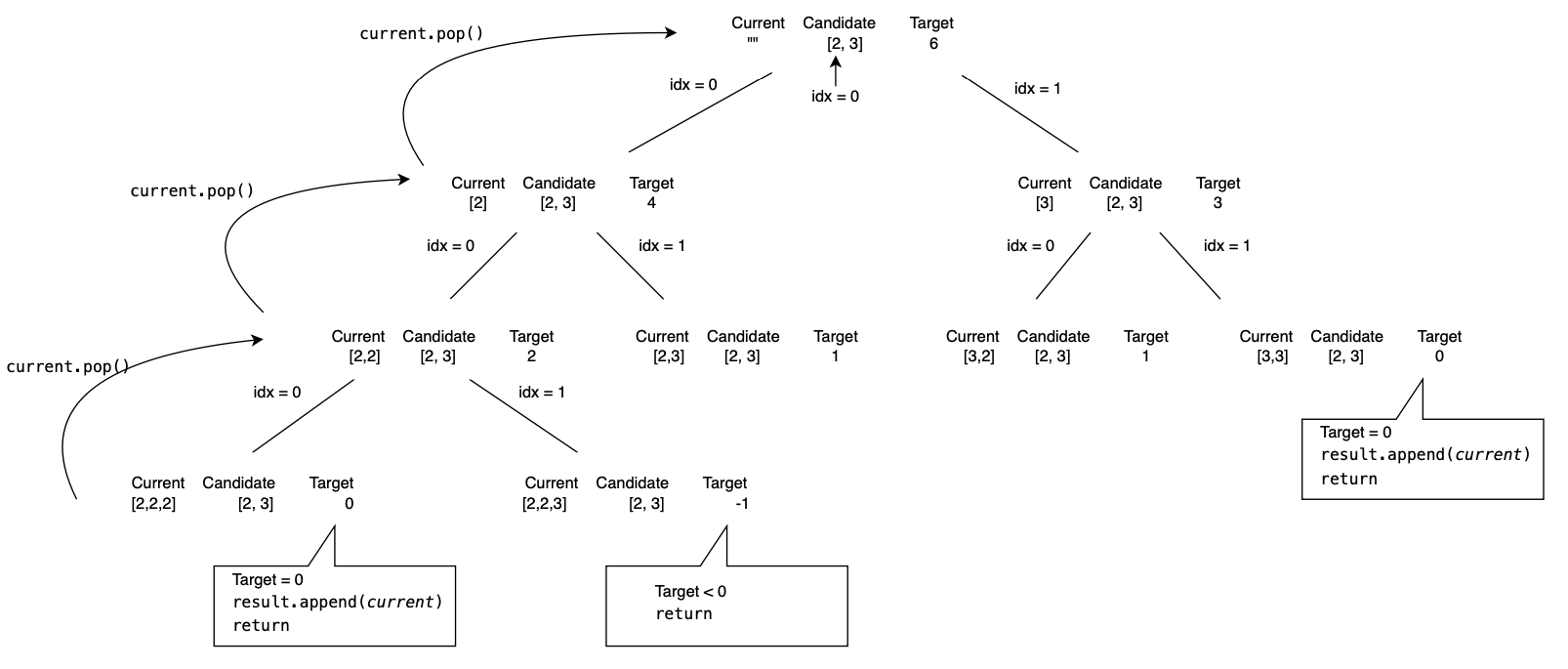# Combination Sum problemIn this article at OpenGenus, we have covered the topic on Solving the Combination Sum problem using Backtracking. We have presented the Time and Space Complexity for various cases.

1. Introduction to Combination Sum problem
2. Solving the Combination Sum problem using Backtracking
3. Time and Space Complexity
4. Conclusion

## Introduction to Combination Sum problem

The Combination Sum problem is defined as follows: Given a set of candidate numbers (without duplicates) and a target number, find all unique combinations in candidates where the candidate numbers sum to target. The same repeated number may be chosen from candidates unlimited number of times.

Inputs:
A list of distinct integers candidates
An integer target representing the sum we want to achieve

Expected Output:
A list of all unique combinations of numbers from candidates that add up to target

Constraints:
1 <= candidates.length <= 30
2 <= candidates[i] <= 40
All elements of candidates are distinct.
1 <= target <= 40

## Solving the Combination Sum problem using Backtracking

``````class Solution(object):
def combination_sum(self, candidates, target):
result = []
current = []
start = 0
self.traverse(result, current, start, target, candidates)
return result
``````

The main function is `combination_sum()`, which is called with a list of `candidate` numbers and a `target` number. It sets up the list of candidates, the current combination, the starting index. The `traverse` function is then called to begin the search for combinations.

``````    def traverse(self, result, current, start, target, candidates):
if target == 0:
result.append(list(current))
return
``````

The function `traverse()` carries out the bulk of the operations. It is a recursive method that explores every possible combination of `candidate` numbers. First it determines if the target equals `0`, which indicates that the sum of the `current` list of numbers matches the initial `target`. If that's the case, it appends the `current` list to the `result` list and then call the `return` statement, which ends the function call and returns the result to the caller.

``````        if target < 0:
return
``````

If the `target` is less than `0`, this suggests that that the `current` combination of numbers sums up to more than the initial `target`. In this case, it immediately call the `return` statement without appending anything to the `result` list.

``````        for idx in range(start, len(candidates)):
current.append(candidates[idx])
self.traverse(result, current, idx, target - candidates[idx], candidates)
current.pop()
``````

If the `target` is still greater than 0, the function loops over the `candidates` starting from the current index. For each `candidate`, it adds the `candidate` to the `current` list and recursively calls the `traverse()` function with the reduced `target` number. This is where the function explores each possible combination.

After the function has explored all combinations starting with the `current` list, the function backtrack by calling `current.pop()` and remove the last added `candidate` from the `current` list.

###### Here is the entire code block for reference
``````class Solution(object):
def combination_sum(self, candidates, target):
result = []
current = []
start = 0
self.traverse(result, current, start, target, candidates)
return result

def traverse(self, result, current, start, target, candidates):
if target == 0:
result.append(list(current))
return
if target < 0:
return
for idx in range(start, len(candidates)):
current.append(candidates[idx])
self.traverse(result, current, idx, target - candidates[idx], candidates)
current.pop()
sol = Solution()
candidates = [2,3]
target = 6
print(sol.combination_sum(candidates, target))
``````
###### Tree DiagramWe can illustrate how the program work by using the tree diagram above. At the top of the tree `combination_sum()` is called to execute the program. We initialise the value of `result`, `current`, `start`, `target` and `candidates` here.

Next the goal test is performed, we checked whether `target = 0` (`current` list is appended to `result` list and the `return` statement is called) or `target < 0` (call the `return` statement without appending anything to the `result` list).

The program then loop through each element of the `candidate` list. It then append the current element of the `candidate` to the `current` list. The program recursive call the `traverse` function and traverse the down the tree in a Depth First Search manner.

Once we reach the bottom of the tree, the program will backtrack one level up the tree by calling `current.pop()` to undo the previous `candidate` selection from the `current` list.

The program end when all possible combination had been explored.

## Time and Space Complexity

The time complexity is O(N^(T/M)) where:

N is the number of candidates,
T is the target value,
M is the minimum value in the candidates.

This is the worst-case scenario, in which we must go over each combination sum individually. This occurs when we have the least number of candidates and the target number is the largest. Backtracking algorithms will try every conceivable combination, which in this case is N^(T/M).

The space complexity is O(T/M). This is because, in the worst-case scenario, adding the smallest number up to the target would need storing T/M elements. This happens when the depth of the recursive call stack reaches its limit.

## Conclusion

One way of improving the efficiency of the program is by sorting the candidate numbers before we solve the problem. This step is not required for solving the problem, but it can help improve efficiency by allowing us to stop the search as soon as the sum of the current combinations exceeds the target.

With this article at OpenGenus, you must have the complete idea of solving the Combination Sum problem using Backtracking.